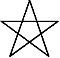Art, History, Music, Politics, and Spirituality For The Modern Alchemist – circulation in over 129 countries

# The Sign of Sheba Year 18,003: Begins June 23rd 2015The Sign of Sheba Year 18,003: Begins June 23 2015

There are eight months in the Calendar of Mu, sometimes referred to as the Nyarzirian Calendar. Each month consists of forty-five days, and represents a system of the eight hexagrams moving through the five elements. The Ninzuwu Priest/Priestess invokes the mudra/mantra formulae associated with each hexagram that rules a particular day after invoking the Armor of Amaterasu Ohkami. The chart below illustrates the daily Vasuh letters invoked and the ruling “deity” for the said hexagram:
1. June 23th, 2015 = Tasuta = 9th Hexagram = Hmu-Bnhu
2. June 24th 2015 = Kukunochi = 20th Hexagram = Hmu-Tuu
3. June 25th 2015 = Ieh = 37th Hexagram = Zhee-Zhee-Lewhu
4. June 26th 2015 = Gzk = 42nd Hexagram = Zhee-Tuu-Hmu-Nzu-Aum
5. June 27th 2015 = Jhn = 53rd Hexagram = Zhee-Bnhu
6. June 28th 2015 = Sheba = 57th Hexagram = Hmu-Shki-Bnhu-Bnhu
7. June 29th 2015 = Lzwa = 59th Hexagram = Aum-Shki
8. June 30th 2015 = Kuhvi = 61st Hexagram = Phe-Phe
9. July 1st 2015 =Entering Earth Element
10. July 2nd2015 = Tasuta = 9th Hexagram = Hmu-Bnhu
11. July 3rd 2015 = Kukunochi = 20th Hexagram = Hmu-Tuu
12. July 4th 2015 = Ieh = 37th Hexagram = Zhee-Zhee-Lewhu
13. July 5th 2015 = Gzk = 42nd Hexagram = Zhee-Tuu-Hmu-Nzu-Aum
14. July 6th 2015 = Jhn = 53rd Hexagram = Zhee-Bnhu
15. July 7th 2015 = Sheba = 57th Hexagram = Hmu-Shki-Bnhu-Bnhu
16. July 8th 2015 = Lzwa = 59th Hexagram = Aum-Shki
17. July 9th 2015 = Kuhvi = 61st Hexagram = Phe-Phe
18. July 10th 2015 =Entering Water Element
19. July 11th 2015 = Tasuta = 9th Hexagram = Hmu-Bnhu
20. July 12th 2015 = Kukunochi = 20th Hexagram = Hmu-Tuu
21. July 13th 2015 = Ieh = 37th Hexagram = Zhee-Zhee-Lewhu
22. July 14th 2015 = Gzk = 42nd Hexagram = Zhee-Tuu-Hmu-Nzu-Aum
23. July 15th 2015 = Jhn = 53rd Hexagram = Zhee-Bnhu
24. July 16th 2015 = Sheba = 57th Hexagram = Hmu-Shki-Bnhu-Bnhu
25. July 17th 2015 = Lzwa = 59th Hexagram = Aum-Shki
26. July 18th 2015 = Kuhvi = 61st Hexagram = Phe-Phe
27. July 19th 2015 =Entering Fire Element
28. July 20th 2015 = Tasuta = 9th Hexagram = Hmu-Bnhu
29. July 21st 2015 = Kukunochi = 20th Hexagram = Hmu-Tuu
30. July 22nd 2015 = Ieh = 37th Hexagram = Zhee-Zhee-Lewhu
31. July 23rd 2015 = Gzk = 42nd Hexagram = Zhee-Tuu-Hmu-Nzu-Aum
32. July 24th 2015 = Jhn = 53rd Hexagram = Zhee-Bnhu
33. July 25th 2015 = Sheba = 57th Hexagram = Hmu-Shki-Bnhu-Bnhu
34. July 26th 2015 = Lzwa = 59th Hexagram = Aum-Shki
35. July 27th 2015 = Kuhvi = 61st Hexagram = Phe-Phe
36. July 28th 2015 =Entering Air Element
37. July 29th 2015 = Tasuta = 9th Hexagram = Hmu-Bnhu
38. July 30th 2015 = Kukunochi = 20th Hexagram = Hmu-Tuu
39. July 31st 2015 = Ieh = 37th Hexagram = Zhee-Zhee-Lewhu
40. August 1st 2015 = Gzk = 42nd Hexagram = Zhee-Tuu-Hmu-Nzu-Aum
41. August 2nd 2015 = Jhn = 53rd Hexagram = Zhee-Bnhu
42. August 3rd 2015 = Sheba = 57th Hexagram = Hmu-Shki-Bnhu-Bnhu
43. August 4th 2015 = Lzwa = 59th Hexagram = Aum-Shki
44. August 5th 2015 = Kuhvi = 61st Hexagram = Phe-Phe
45. August 6th 2015 =Entering Void Element

#### You may have missed#### One Breath Away by Radio Drive – An Astonishing EP#### The Blackbird Sessions by Sugar Lime Blue ( Album Review)